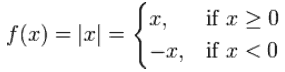# Absolute Value Function

This is the Absolute Value Function:

f(x) = |x|

It is also sometimes written: abs(x)

This is its graph:f(x) = |x|

It makes a right angle at (0,0)

It is an even function.

Its Domain is the Real Numbers:Its Range is the Non-Negative Real Numbers: [0, +∞)

Are you absolutely positive? Yes! Except when I am zero.

## Piecewise

It is also a Piecewise Function:Plot the graph here